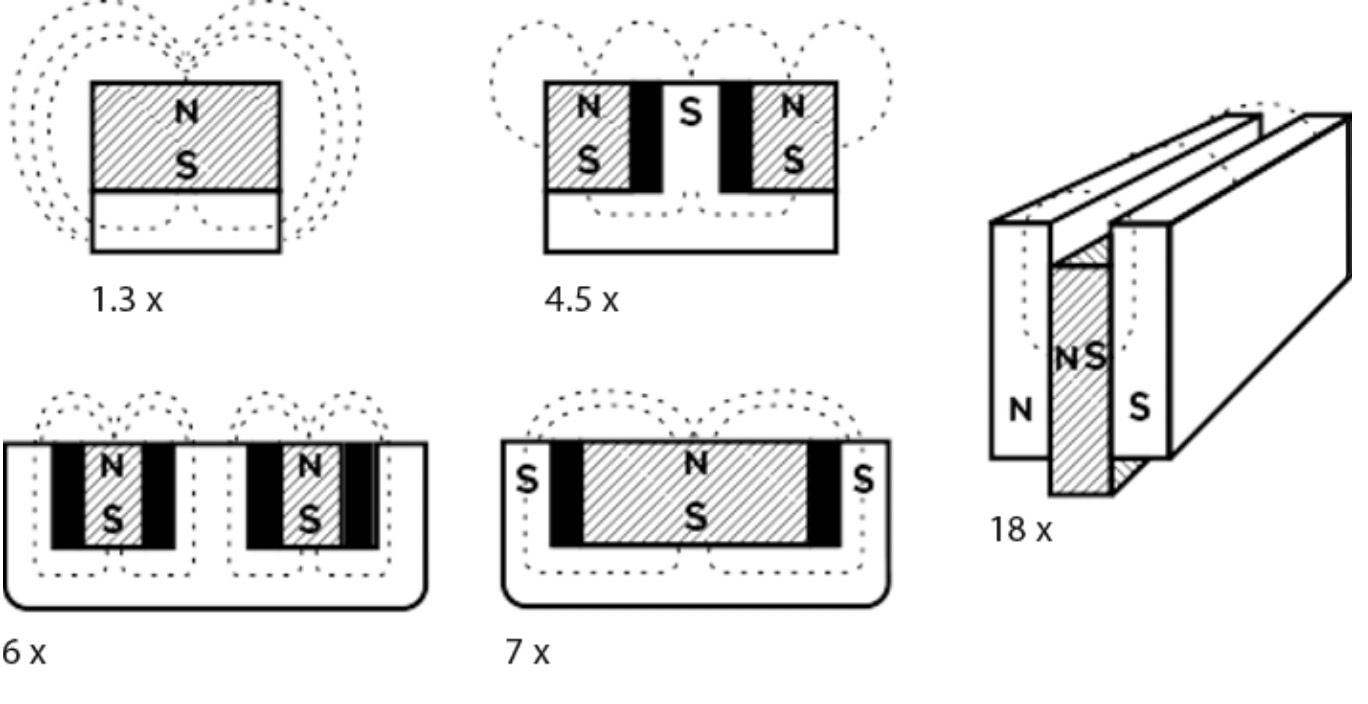# What is magnetism?

Magnetism is a phenomenon in which one material exerts forces of attraction on other materials.

## Lasting strengths

Neodymium magnets are strong magnets that retain their enormous force without notable weakening. This is irrespective of whether the magnet is being used to lift something for years at a time or not used at all. Generally, the force weakens at a rate of approx. 10% every 100 years.Turbocharged power

## The same magnet but much stronger

Using iron and/or steel in combination with a magnet can enhance the magnetic force. This creates what is known as a magnetic system.

The illustrations give an idea of how much stronger the magnetic system is than if the magnet in the magnetic system were used on its own. Of course, the right design is necessary for this to work.

Remember that magnetic systems are very sensitive to air gaps!

Units

## Gauss & Tesla

The table contains some important magnetic terms from both the Gauss and SI systems. The Gauss system is still used for permanent magnets.

Please note that magnetic flux (Br), which can be found in the data sheets, is not the same as the value that can be measured at the surface of the magnet using a gaussmeter.

For example, a NdFeB magnet Ø20x10 mm with Br = 12,000 Gauss (as per spec.) produces a value of approx. 3,000 Gauss at the surface of the magnet.

• 1 T = 10,000 G (The Earth’s magnetic field is approx. 0.5 Gauss)
• 1 kA/m = 12.57 Oe
• 1 kJ/m³ = 0.1257 MGOe
 Quantity Symbol Unit (Gauss) Unit (SI) Field strength H Oerstedt (Oe) Ampere / meter (A/m) Flux density B Gauss (G) Tesla (T), Vs/m² Magnetic flux Φ Maxwell (Mx) Weber (Wb), Vs Energy product (BH)max MGOe J/m³